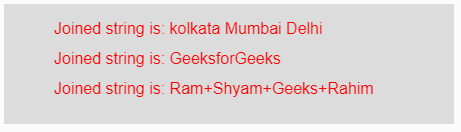# p5.js | join() function

The join() function in p5.js is used to join the input array of strings into a single string using separator. This separator might be any character which gets their position in between two strings of the input array.

Syntax:

`join(Array, Separator)`

Parameters: This function accepts two parameters as mentioned above and described below:

• Array: This is the array of strings which are to be joined.
• Separator: This is any character to be placed in between two strings of the input array.

Return Value: It returns the joined strings.

Below programs illustrate the join() function in p5.js:

Example 1: This example uses join() function to join the input array elements.

 `function` `setup() {  ` `  `  `    ``// Creating Canvas size ` `    ``createCanvas(450, 120);  ` `}  ` `  `  `function` `draw() {  ` `      `  `    ``// Set the background color  ` `    ``background(220);  ` `    `  `    ``// Initializing the Array of strings ` `    ``let Array1 = [``"Geeks"``, ``"for"``, ``"Geeks"``]; ` `    ``let Array2 = [``"1"``, ``"2"``, ``"3"``]; ` `    ``let Array3 = [``"a"``, ``"A"``, ``"b"``, ``"B"``]; ` `    `  `    ``// Initializing the separator character ` `    ``let Separator1 = ``"/"``; ` `    ``let Separator2 = ``"&"``; ` `    ``let Separator3 = ``"*"``; ` `          `  `    `  `    ``// Calling to join() function. ` `    ``let A = join(Array1, Separator1); ` `    ``let B = join(Array2, Separator2); ` `    ``let C = join(Array3, Separator3); ` `          `  `    ``// Set the size of text  ` `    ``textSize(16);  ` `      `  `    ``// Set the text color  ` `    ``fill(color(``'red'``));  ` `    `  `    ``// Getting joined strings ` `    ``text(``"Joined string is: "` `+ A, 50, 30); ` `    ``text(``"Joined string is: "` `+ B, 50, 60); ` `    ``text(``"Joined string is: "` `+ C, 50, 90); ` `       `  `}  `

Output:Example 2: This example uses join() function to join the input array elements.

 `function` `setup() {  ` `  `  `    ``// Creating Canvas size ` `    ``createCanvas(450, 120);  ` `}  ` `  `  `function` `draw() {  ` `      `  `    ``// Set the background color  ` `    ``background(220); ` `          `  `    ``// Calling join() function over the parameter ` `    ``// Array of strings and separator ` `    ``let A = join([``"kolkata"``, ``"Mumbai"``, ``"Delhi"``], ``" "``); ` `    ``let B = join([``"Geeks"``, ``"for"``, ``"Geeks"``], ``""``); ` `    ``let C = join([``"Ram"``, ``"Shyam"``, ``"Geeks"``, ``"Rahim"``], ``"+"``); ` `          `  `    ``// Set the size of text  ` `    ``textSize(16);  ` `      `  `    ``// Set the text color  ` `    ``fill(color(``'red'``));  ` `    `  `    ``// Getting joined strings ` `    ``text(``"Joined string is: "` `+ A, 50, 30); ` `    ``text(``"Joined string is: "` `+ B, 50, 60); ` `    ``text(``"Joined string is: "` `+ C, 50, 90); ` `}  `

Output:Reference: https://p5js.org/reference/#/p5/join

My Personal Notes arrow_drop_upCheck out this Author's contributed articles.

If you like GeeksforGeeks and would like to contribute, you can also write an article using contribute.geeksforgeeks.org or mail your article to contribute@geeksforgeeks.org. See your article appearing on the GeeksforGeeks main page and help other Geeks.

Please Improve this article if you find anything incorrect by clicking on the "Improve Article" button below.

Article Tags :

Be the First to upvote.

Please write to us at contribute@geeksforgeeks.org to report any issue with the above content.